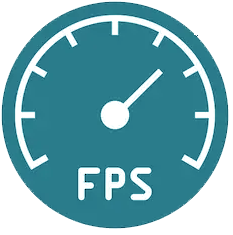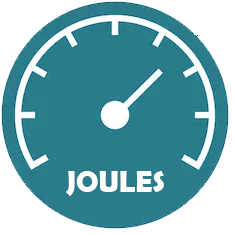FPS/Joules Calculator

 How to use the FPS and Velocity Calculator   Enter your desired BB weight and Speed under "Input 1" and click calculate. This will show you under "Results 1" the corosponding velocity in meters per second and energy in joules.   How to use the Range Calculator Enter the Energy (Joule) results from the FPS and Velocity calculator in the "Input 2" field along with your BB weight in grams. Click calculate, this will show you under Results 2 the aproximate Muzzle Velocity in FPS and Meters Per Second and Range in Feet and Meters for your Airsoft Gun.       The "Velocity (m/s)" results can also be used for determining which spring to select for a desired FPS. For example entering 400 in the "Speed(FPS)" box under "Input 1" and clicking "Calculate" will give you a result of 121.6 in the "Velocity(m/s) Results 1", as most Airsoft spring rate designations are based on Meters Per Second (M) we can now round the result and determine in order to achieve 400FPS we would need an M120 spring. (Other internal components may effect performance but it will give you a good starting point) The calculator "Range" results are based on shooting an Airsoft Gun with a minimum barrel length of 300mm in a level possition at 3 feet above the ground, the resulting "Range" calculation indicates the end of travel where the BB contacts the ground. The calculator does not take into account other external factors such as wind which may effect range and accuracy. Input 1 BB weight(Grams): Speed(FPS):Results 1 Velocity (m/s): Energy (Joule):         Input 2 BB weight(Grams): Energy(Joule):Results 2 Muzzle Velocity Speed(FPS): Velocity(m/s): Estimated Range Feet: Meters:×
×

Vendor:
View full product info

-->